# Samacheer Kalvi 12th Maths Solutions Chapter 11 Probability Distributions Ex 11.6

## Tamilnadu Samacheer Kalvi 12th Maths Solutions Chapter 11 Probability Distributions Ex 11.6

Choose the Correct or the most suitable answer from the given four alternatives:

Question 1.
Let X be random variable with probability density function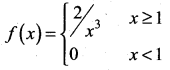Which of the following statement is correct
(a) both mean and variance exist
(b) mean exists but variance does not exist
(c) both mean and variance do not exist
(d) variance exists but Mean does not exist
Solution:
(b) mean exists but variance does not exist
Hint: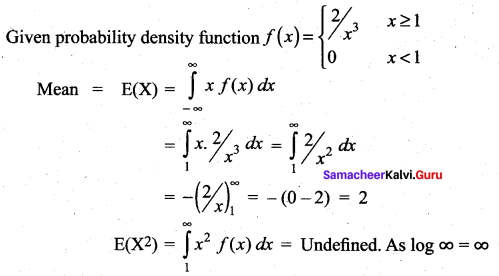Question 2.
A rod of length 21 is broken into two pieces at random. The probability density function of the shorter of the two pieces is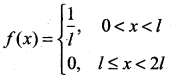The mean and variance of the shorter of the two pieces are respectively ……..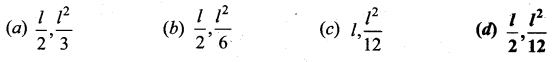Solution:
(d) $$\frac{l}{2}, \frac{l^{2}}{12}$$
Hint: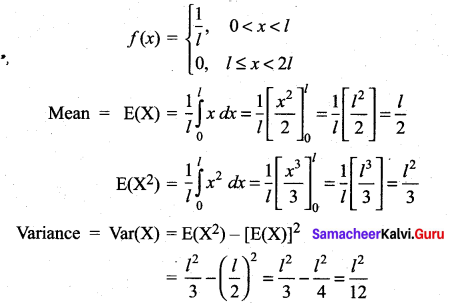Question 3.
Consider a game where the player tosses a six-sided fair die. If the face that comes up is 6, the player wins ₹ 36, otherwise he loses ₹ k2, where k is the face that comes up k = {1, 2, 3, 4, 5}. The expected amount to win at this game in ₹ is ……..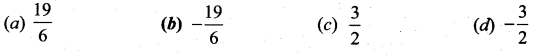Solution:
(b) $$-\frac{19}{6}$$
Hint: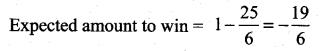Question 4.
A pair of dice numbered 1, 2, 3, 4, 5, 6 of a six-sided die and 1, 2, 3, 4 of a four-sided die is rolled and the sum is determined. Let the random variable X denote this sum. Then the number of elements in the inverse image of 7 is ………
(a) 1
(b) 2
(c) 3
(d) 4
Solution:
(d) 4
Hint:
Sample points to get the sum ‘7’ are (3, 4), (4, 3), (5, 2), (6, 1)
Number of elements in inverse image of 7 = 4

Question 5.
A random variable X has binomial distribution with n = 25 and p = 0.8 then standard deviation of X is ……
(a) 6
(b) 4
(c) 3
(d) 2
Solution:
(d) 2
Hint:
n = 25, p = 0.8, ∴ q = 1 – 0.8 = 0.2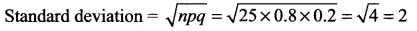Question 6.
Let X represent the difference between the number of heads and the number of tails obtained when a coin is tossed n times. Then the possible values of X are
(a) i + 2n, i = 0, 1, 2 … n
(b) 2i – n, i = 0, 1, 2 … n
(c) n – i, i = 0, 1, 2 … n
(d) 2i + 2n, i = 0, 1, 2 … n
Solution:
(b) 2i – n, i = 0, 1, 2 … n

Question 7.
If the function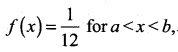represents a probability density function of a continuous random variable X, then which of the following cannot be the value of a and b?
(a) 0 and 12
(b) 5 and 17
(c) 7 and 19
(d) 16 and 24
Solution:
(d) 16 and 24
Hint: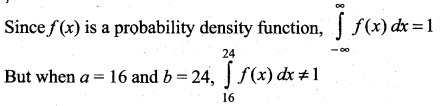Question 8.
Four buses carrying 160 students from the same school arrive at a football stadium. The buses carry, respectively, 42, 36, 34, and 48 students. One of the students is randomly selected. Let X denote the number of students that were on the bus carrying the randomly selected student. One of the 4 bus drivers is also randomly selected. Let Y denote the number of students on that bus. Then E[X] and E[Y] respectively are
(a) 50, 40
(b) 40, 50
(c) 40.75, 40
(d) 41,41
Solution:
(c) 40.75, 40
Hint:
E[X] > E[Y], Since the students selected is more likely to come from the bus carrying maximum number of students whereas the drivers are equally likely to be selected.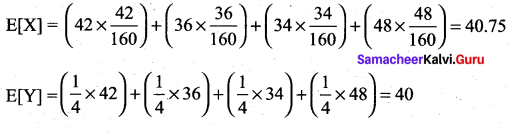Question 9.
Two coins are to be flipped. The first coin will land on heads with probability 0.6, the second with Probability 0.5. Assume that the results of the flips are independent, and let X equal the total number of heads that result. The value of E[X] is …..
(a) 0.11
(b) 1.1
(c) 11
(d) 1
Solution:
(b) 1.1
Hint:
P (X = 0) = P (TT)
= P (T) P (T) = (0.4) (0.5) = 0.20
P (X = 1) = P (HT) + P(TH) = (0.6) (0.5) + (0.4) (0.5) = 0.5
P (X = 2) = P (HH)
= P (H). P (H) = (0.6) (0.5) = 0.30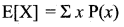= (0).(0.20) + (1) (0.5) + (2) (0.30)
= 0.5 + 0.6 = 1.1Question 10.
On a multiple-choice exam with 3 possible destructives for each of the 5 questions, the probability that a student will get 4 or more correct answers just by guessing is …….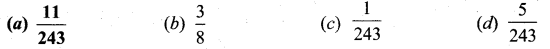Solution:
(a) $$\frac{11}{243}$$
Hint: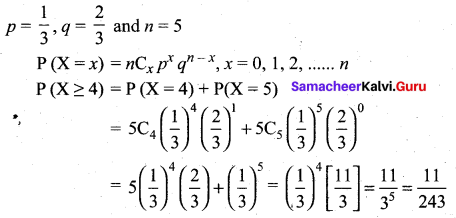Question 11.
If P{X = 0} = 1 – P{X = 1}. If E[X] = 3Var(X), then P{X = 0}.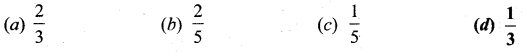Solution:
(d) $$\frac{1}{3}$$
Hint:
Given P (X = 0) = 1 – P (X = 1) ⇒ P (X = 1) = 1 – P (X = 0)
Let n = P(X = 0), ∴ P(X = 1) = 1 -n
E(X) = (0) (n) + (1) (1 – n) = 1 – n
E(X2) = (0)2 (n) + (1)2 (1 – n) = 1 – n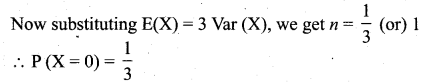Question 12.
If X is a binomial random variable with expected value 6 and variance 2.4, Then P{X = 5} is ……….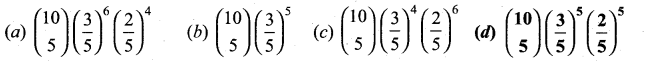Solution:
(d) $$\left(\begin{array}{c}{10} \\ {5}\end{array}\right)\left(\frac{3}{5}\right)^{5}\left(\frac{2}{5}\right)^{5}$$
Hint: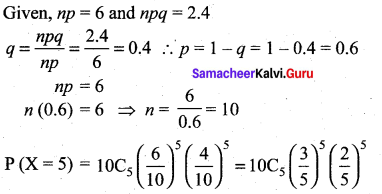Question 13.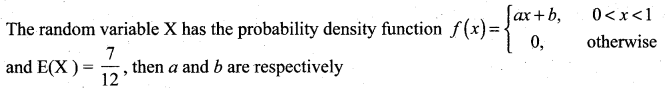(a) 1 and $$\frac{1}{2}$$
(b) $$\frac{1}{2}$$ and 1
(c) 2 and 1
(d) 1 and 2
Solution:
(a) 1 and $$\frac{1}{2}$$
Hint: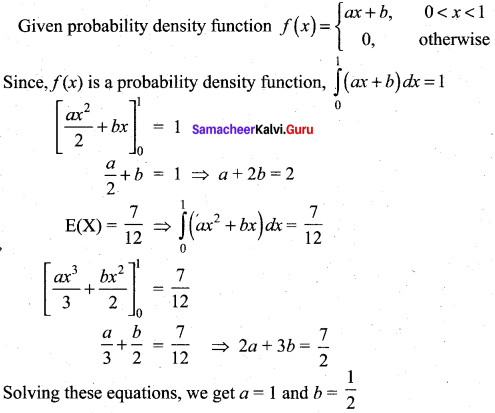Question 14.
Suppose that X takes on one of the values 0, 1, and 2. If for some constant k,
P(X = i) = k P(X = i – 1) for i = 1, 2 P(X = 0) = $$\frac{1}{7}$$. Then the value of k is …..
(a) 1
(b) 2
(c) 3
(d) 4
Solution:
(b) 2
Hint:
P(X = i) = k P(X = i – 1) for i = 1, 2 and P(X = 0) = $$\frac{1}{7}$$
Solving the equation, we get k = 2

Question 15.
Which of the following is a discrete random variable?
I. The number of cars crossing a particular signal in a day.
II. The number of customers in a queue to buy train tickets at a moment.
III. The time taken to complete a telephone call.
(a) I and II
(b) II only
(c) III only
(d) II and III
Solution:
(a) I and II

Question 16.
If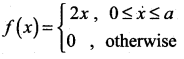is a probability density function of a random variable, then the value of a is ……..
(a) 1
(b) 2
(c) 3
(d) 4
Solution:
(a) 1
Hint: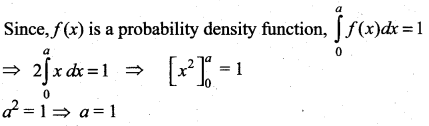Question 17.
The probability function of a random variable is defined as ……..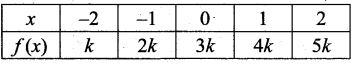Then E(X) is equal to …….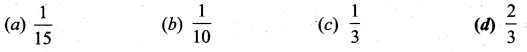Solution:
(d) $$\frac{2}{3}$$
Hint: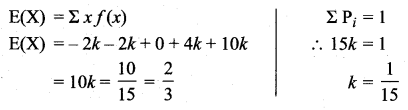Question 18.
Let X have a Bernoulli distribution with mean 0.4, then the variance of (2X – 3) is ……
(a) 0.24
(b) 0.48
(c) 0.6
(d) 0.96
Solution:
(d) 0.96
Hint:
Mean = 0.4 ⇒ µ = p = 0.4
Var (2X – 3) = 22 Var X
= 4(pq) = 4(0.4)(0.6) = 0.96

Question 19.
If in 6 trials, X is a binomial variate which follows the relation 9P(X = 4) = P(X = 2), then the probability of success is …..
(a) 0.125
(b) 0.25
(c) 0.375
(d) 0.75
Solution:
(b) 0.25
Hint: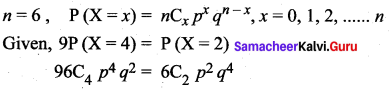9p2 = q2 [∵ 6C4 = 6C2]
9p2 = (1 – p)2
9p2 = 1 – 2p+ p2
8p2 + 2p – 1 = 0
(4p – 1)(2p + 1) = 0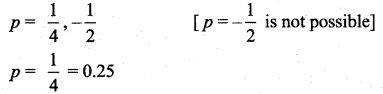Question 20.
A computer salesperson knows from his past experience that he sells computers to one in every twenty customers who enter the showroom. What is the probability that he will sell a computer to exactly two of the next three customers?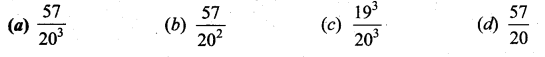Solution:
(a) [ltaex]\frac{57}{20^{3}}[/latex]
Hint: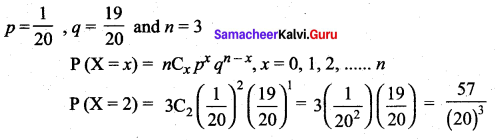### Samacheer Kalvi 12th Maths Solutions Chapter 11 Probability Distributions Ex 11.6 Additional Questions Solved

Choose the Correct or the most suitable answer from the given four alternatives:

Question 1.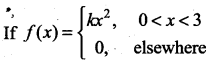is a probability density function then the value of k is ……..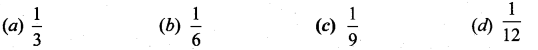Solution:
(c) $$\frac{1}{9}$$
Hint:
Given that f(x) is a probability density function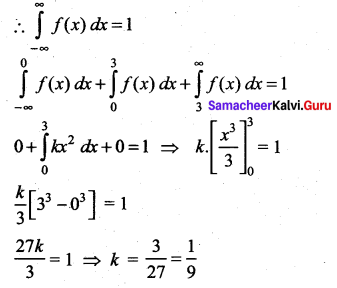Question 2.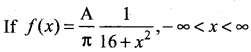is a probability density function of a continuous random variable X, then the value of A is …………
(a) 16
(b) 8
(c) 4
(d) 1
Solution:
(c) 4
Hint: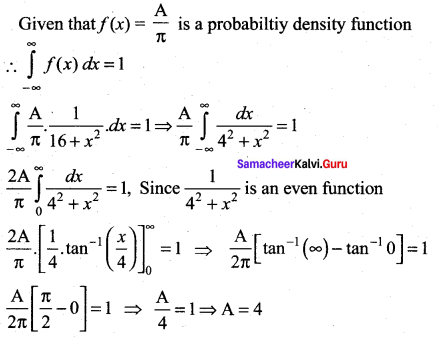Question 3.
A random variable X has the following probability mass function as follows: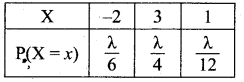Then the value of λ is ……..
(a) 1
(b) 2
(c) 3
(d) 4
Solution:
(b) 2
Hint: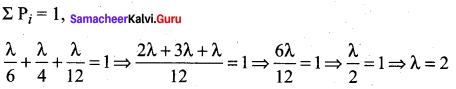Question 4.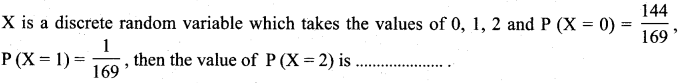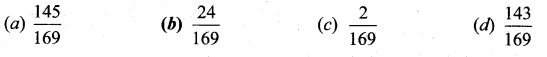Solution:
(b) $$\frac{24}{169}$$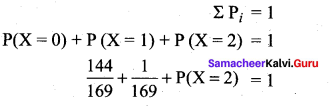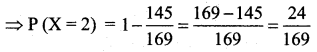Question 5.
Given E(x + c) = 8 and E(x – c) = 12, then the value of c is …….
(a) -2
(b) 4
(c) -4
(d) 2
Solution:
(a) -2
Hint:
E(X + c) = 8
E(X) + E(c) = 8
E(X) + c = 8 ….. (1)
E(X – c) = 12
E(X) – E(c) = 12 ⇒ E(X) – c = 12 ……… (2)
(1) – (2) ⇒ 2c = -4 = c = -2

Question 6.
X is random variable taking the values 3, 4 and 12 with probabilities $$\frac{1}{3}$$, $$\frac{1}{4}$$ and $$\frac{5}{12}$$. Then E(X) is ……..
(a) 5
(b) 7
(c) 6
(d) 3
Solution:
(b) 7
Hint: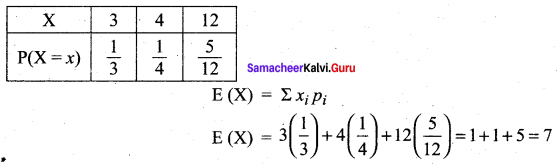Question 7.
Variance of the random variable X is 4. Its mean is 2. Then E(X2) is ……..
(a) 2
(b) 4
(c) 6
(d) 8
Solution:
(d) 8
Hint:
Given, Var (X) = 4
E(X2) – [E(X)]2 = 4
E(X2) – 22 = 4 ⇒ E(X2) = 4 + 4 = 8

Question 8.
In 5 throws of a die, getting 1 or 2 is a success. The mean number of success is ……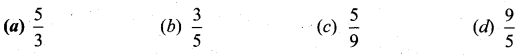Hint:
Given, n = 5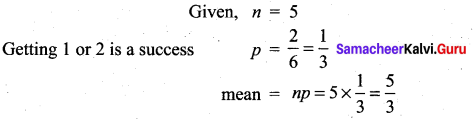Question 9.
The mean of a binomial distribution is 5 and its standard deviation is 2. Then the value of n and p are ……..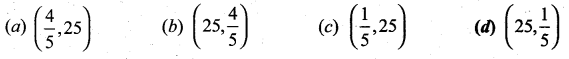Solution:
(d) $$\left(25, \frac{1}{5}\right)$$
Hint: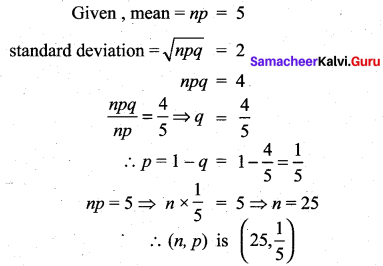Question 10.
If the mean and standard deviation of a binomial distribution are 12 and 2 respectively. Then the value of its parameter p is ……Solution:
(c) $$\frac{2}{3}$$
Hint: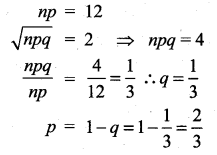Question 11.
A box contains 6 red and 4 white balls. If 3 balls are drawn at random, the probability of getting 2 white balls without replacement is ………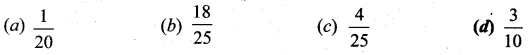Solution:
(d) $$\frac{3}{10}$$
Hint:
Total number of balls = 10
Number of ways of choosing 3 balls from 10 balls = 10C3
Number of ways of choosing 2 white balls from 4 white balls = 4C3
Number of ways of choosing 1 red ball from 6 red balls = 6C1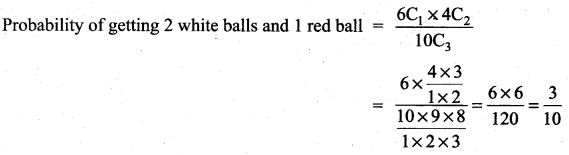Question 12.
If 2 cards are drawn from a well shuffled pack of 52 cards, the probability that they are of the same colours without replacement is ………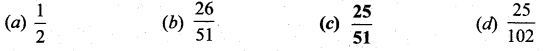Solution:
(c) $$\frac{25}{51}$$
Hint: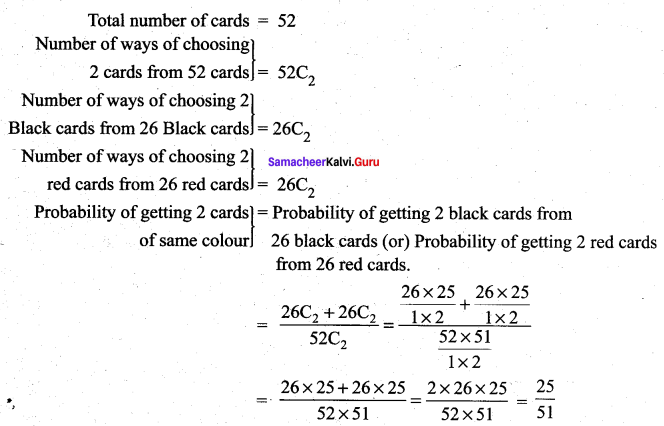Question 13.
The distribution function F(X) of a random variable X is ……..
(a) a decreasing function
(b) a non-decreasing function
(c) a constant function
(d) increasing first and then decreasing
Solution:
(b) a non-decreasing function